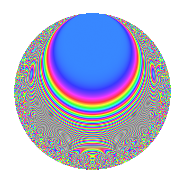# Properties

 Label 525.2.gLevel 525 Weight 2 Character orbit g Rep. character $$\chi_{525}(524,\cdot)$$ Character field $$\Q$$ Dimension 44 Newforms 6 Sturm bound 160 Trace bound 6

# Learn more about

## Defining parameters

 Level: $$N$$ = $$525 = 3 \cdot 5^{2} \cdot 7$$ Weight: $$k$$ = $$2$$ Character orbit: $$[\chi]$$ = 525.g (of order $$2$$ and degree $$1$$) Character conductor: $$\operatorname{cond}(\chi)$$ = $$105$$ Character field: $$\Q$$ Newforms: $$6$$ Sturm bound: $$160$$ Trace bound: $$6$$ Distinguishing $$T_p$$: $$2$$, $$41$$

## Dimensions

The following table gives the dimensions of various subspaces of $$M_{2}(525, [\chi])$$.

Total New Old
Modular forms 92 52 40
Cusp forms 68 44 24
Eisenstein series 24 8 16

## Trace form

 $$44q$$ $$\mathstrut +\mathstrut 48q^{4}$$ $$\mathstrut +\mathstrut 8q^{9}$$ $$\mathstrut +\mathstrut O(q^{10})$$ $$44q$$ $$\mathstrut +\mathstrut 48q^{4}$$ $$\mathstrut +\mathstrut 8q^{9}$$ $$\mathstrut +\mathstrut 40q^{16}$$ $$\mathstrut -\mathstrut 18q^{21}$$ $$\mathstrut -\mathstrut 24q^{36}$$ $$\mathstrut -\mathstrut 48q^{39}$$ $$\mathstrut -\mathstrut 88q^{46}$$ $$\mathstrut +\mathstrut 34q^{49}$$ $$\mathstrut -\mathstrut 60q^{51}$$ $$\mathstrut -\mathstrut 32q^{64}$$ $$\mathstrut +\mathstrut 8q^{79}$$ $$\mathstrut +\mathstrut 112q^{81}$$ $$\mathstrut -\mathstrut 36q^{84}$$ $$\mathstrut +\mathstrut 54q^{91}$$ $$\mathstrut +\mathstrut 4q^{99}$$ $$\mathstrut +\mathstrut O(q^{100})$$

## Decomposition of $$S_{2}^{\mathrm{new}}(525, [\chi])$$ into irreducible Hecke orbits

Label Dim. $$A$$ Field CM Traces $q$-expansion
$$a_2$$ $$a_3$$ $$a_5$$ $$a_7$$
525.2.g.a $$4$$ $$4.192$$ $$\Q(\zeta_{12})$$ $$\Q(\sqrt{-3})$$ $$0$$ $$0$$ $$0$$ $$0$$ $$q-\zeta_{12}q^{3}-2q^{4}+(\zeta_{12}-\zeta_{12}^{2})q^{7}+\cdots$$
525.2.g.b $$4$$ $$4.192$$ $$\Q(\zeta_{12})$$ None $$0$$ $$0$$ $$0$$ $$0$$ $$q+\zeta_{12}q^{2}-\zeta_{12}q^{3}+q^{4}-3q^{6}+(-\zeta_{12}+\cdots)q^{7}+\cdots$$
525.2.g.c $$4$$ $$4.192$$ $$\Q(\zeta_{12})$$ None $$0$$ $$0$$ $$0$$ $$0$$ $$q-\zeta_{12}q^{2}-\zeta_{12}q^{3}+q^{4}+3q^{6}+(-\zeta_{12}+\cdots)q^{7}+\cdots$$
525.2.g.d $$8$$ $$4.192$$ 8.0.303595776.1 None $$0$$ $$0$$ $$0$$ $$0$$ $$q+(\beta _{1}-\beta _{4}-\beta _{5})q^{2}-\beta _{1}q^{3}+(1-\beta _{2}+\cdots)q^{4}+\cdots$$
525.2.g.e $$8$$ $$4.192$$ 8.0.303595776.1 None $$0$$ $$0$$ $$0$$ $$0$$ $$q-\beta _{6}q^{2}+(-\beta _{2}-\beta _{4}-\beta _{6})q^{3}+(1+\cdots)q^{4}+\cdots$$
525.2.g.f $$16$$ $$4.192$$ $$\mathbb{Q}[x]/(x^{16} + \cdots)$$ None $$0$$ $$0$$ $$0$$ $$0$$ $$q+\beta _{2}q^{2}+\beta _{4}q^{3}+(1-\beta _{5})q^{4}-\beta _{8}q^{6}+\cdots$$

## Decomposition of $$S_{2}^{\mathrm{old}}(525, [\chi])$$ into lower level spaces

$$S_{2}^{\mathrm{old}}(525, [\chi]) \cong$$ $$S_{2}^{\mathrm{new}}(105, [\chi])$$$$^{\oplus 2}$$